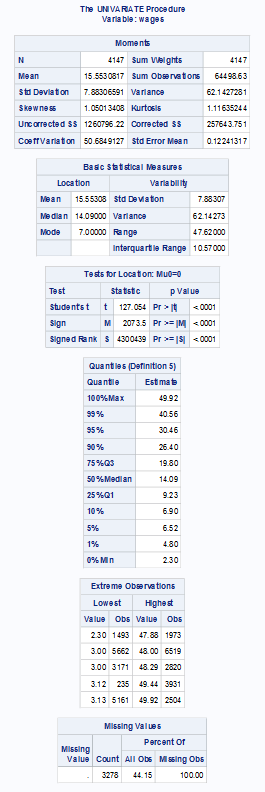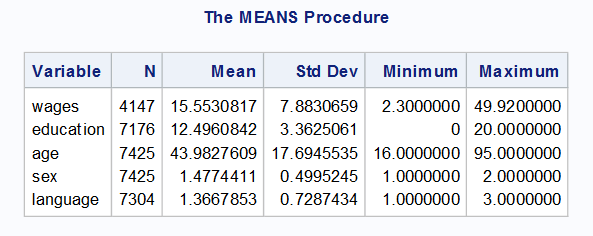It looks like you're using Internet Explorer 11 or older. This website works best with modern browsers such as the latest versions of Chrome, Firefox, Safari, and Edge. If you continue with this browser, you may see unexpected results.

# SAS

## Descriptive Statistics for One Variable

Getting the descriptive statistics in Sas is quick for one or multiple variables. Descriptive statistics are measures we can use to learn more about the distribution of observations in variables for analysis, transforming variables, and reporting. Each descriptive statistic has their own formula that we will not be covering in this guide, but we will walk through the interpretation of each.

Below is the code for calculating the descriptive statistics of the variable wages.

Code

PROC UNIVARIATE DATA = SLID;
VAR wages;
RUN

We are conducting a PROC (procedure) to calculate the UNIVARIATE (descriptive statistics) for the VAR (variable) wages in the DATA SLID. We then end with the RUN command.

OutputA

The output chart shows us a host of information about the variable wages including descriptive statistics, data information, specific observations, and missing values. We are going to focus on a couple of descriptive statistics on this output.

The second chart, Moments, shows us the descriptive statistics and other measures. We can on the first row to the left, see under the column there are 4,147 observations without missing data for the variable wages.

Below that the average wage value in this dataset is 15.5530817 which is below the middle value of 26.11 ((49.92 – 2.30)/2) (not shown), indicating the distribution of the data is skewed toward lower values. We also have the kurtosis and variance calculated for us in the first chart.

The standard deviation is 7.88306591, indicating there is a wide distribution of the data.

## Descriptive Statistics for Multiple Variables

We can also calculate the descriptive statistics for all the variables in one command line.

Code

PROC MEANS DATA = SLID;
RUN
;

With this code we are conducting the PROC (procedure) to calculate the MEANS (descriptive statistics) for all the variables in the DATA SLID. We then end with the RUN command.

OutputIn this chart Sas provides us with each variable name, the number of observations, the mean, standard deviation, the minimum, and the maximum values for each.